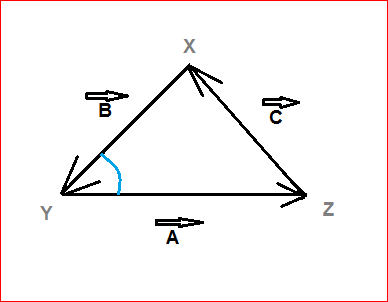# Vector Basics

Geometry Level 2If triangle $XYZ$ is an equilateral triangle, then, what is the measure of the angle (in degrees) between the vectors $\vec { A }$ and $\vec { B }$ in the figure above.

×

Problem Loading...

Note Loading...

Set Loading...